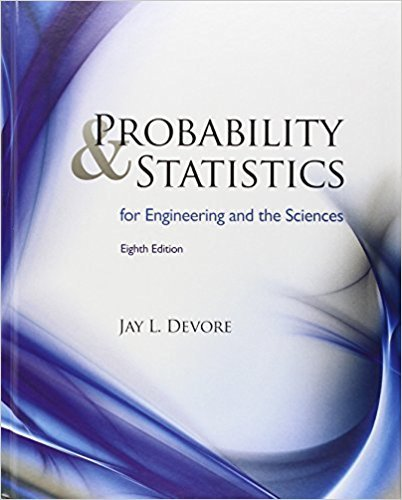×
×

# Solutions for Chapter 1: Overview and Descriptive Statistics## Full solutions for Probability and Statistics for Engineering and the Sciences | 8th Edition

ISBN: 9780538733526Solutions for Chapter 1: Overview and Descriptive Statistics

Solutions for Chapter 1
4 5 0 253 Reviews
25
0
##### ISBN: 9780538733526

Chapter 1: Overview and Descriptive Statistics includes 165 full step-by-step solutions. Since 165 problems in chapter 1: Overview and Descriptive Statistics have been answered, more than 29048 students have viewed full step-by-step solutions from this chapter. Probability and Statistics for Engineering and the Sciences was written by and is associated to the ISBN: 9780538733526. This expansive textbook survival guide covers the following chapters and their solutions. This textbook survival guide was created for the textbook: Probability and Statistics for Engineering and the Sciences , edition: 8.

Key Statistics Terms and definitions covered in this textbook
• `-error (or `-risk)

In hypothesis testing, an error incurred by rejecting a null hypothesis when it is actually true (also called a type I error).

• All possible (subsets) regressions

A method of variable selection in regression that examines all possible subsets of the candidate regressor variables. Eficient computer algorithms have been developed for implementing all possible regressions

• Analysis of variance (ANOVA)

A method of decomposing the total variability in a set of observations, as measured by the sum of the squares of these observations from their average, into component sums of squares that are associated with speciic deined sources of variation

• Assignable cause

The portion of the variability in a set of observations that can be traced to speciic causes, such as operators, materials, or equipment. Also called a special cause.

• Asymptotic relative eficiency (ARE)

Used to compare hypothesis tests. The ARE of one test relative to another is the limiting ratio of the sample sizes necessary to obtain identical error probabilities for the two procedures.

• Attribute

A qualitative characteristic of an item or unit, usually arising in quality control. For example, classifying production units as defective or nondefective results in attributes data.

• Axioms of probability

A set of rules that probabilities deined on a sample space must follow. See Probability

• Bimodal distribution.

A distribution with two modes

• Categorical data

Data consisting of counts or observations that can be classiied into categories. The categories may be descriptive.

• Central tendency

The tendency of data to cluster around some value. Central tendency is usually expressed by a measure of location such as the mean, median, or mode.

• Chi-square (or chi-squared) random variable

A continuous random variable that results from the sum of squares of independent standard normal random variables. It is a special case of a gamma random variable.

• Continuous distribution

A probability distribution for a continuous random variable.

• Control chart

A graphical display used to monitor a process. It usually consists of a horizontal center line corresponding to the in-control value of the parameter that is being monitored and lower and upper control limits. The control limits are determined by statistical criteria and are not arbitrary, nor are they related to speciication limits. If sample points fall within the control limits, the process is said to be in-control, or free from assignable causes. Points beyond the control limits indicate an out-of-control process; that is, assignable causes are likely present. This signals the need to ind and remove the assignable causes.

• Correction factor

A term used for the quantity ( / )( ) 1 1 2 n xi i n ? = that is subtracted from xi i n 2 ? =1 to give the corrected sum of squares deined as (/ ) ( ) 1 1 2 n xx i x i n ? = i ? . The correction factor can also be written as nx 2 .

• Cumulative distribution function

For a random variable X, the function of X deined as PX x ( ) ? that is used to specify the probability distribution.

• Decision interval

A parameter in a tabular CUSUM algorithm that is determined from a trade-off between false alarms and the detection of assignable causes.

• Extra sum of squares method

A method used in regression analysis to conduct a hypothesis test for the additional contribution of one or more variables to a model.

• First-order model

A model that contains only irstorder terms. For example, the irst-order response surface model in two variables is y xx = + ?? ? ? 0 11 2 2 + + . A irst-order model is also called a main effects model

• Fisher’s least signiicant difference (LSD) method

A series of pair-wise hypothesis tests of treatment means in an experiment to determine which means differ.

• Gaussian distribution

Another name for the normal distribution, based on the strong connection of Karl F. Gauss to the normal distribution; often used in physics and electrical engineering applications

×